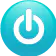# Control Position of DC Motor via Web Using PID Controller

This project shows how to control the position of DC motor via Web using a PID controller.

BeginnerFull instructions provided4,162

## Things used in this project

### Hardware components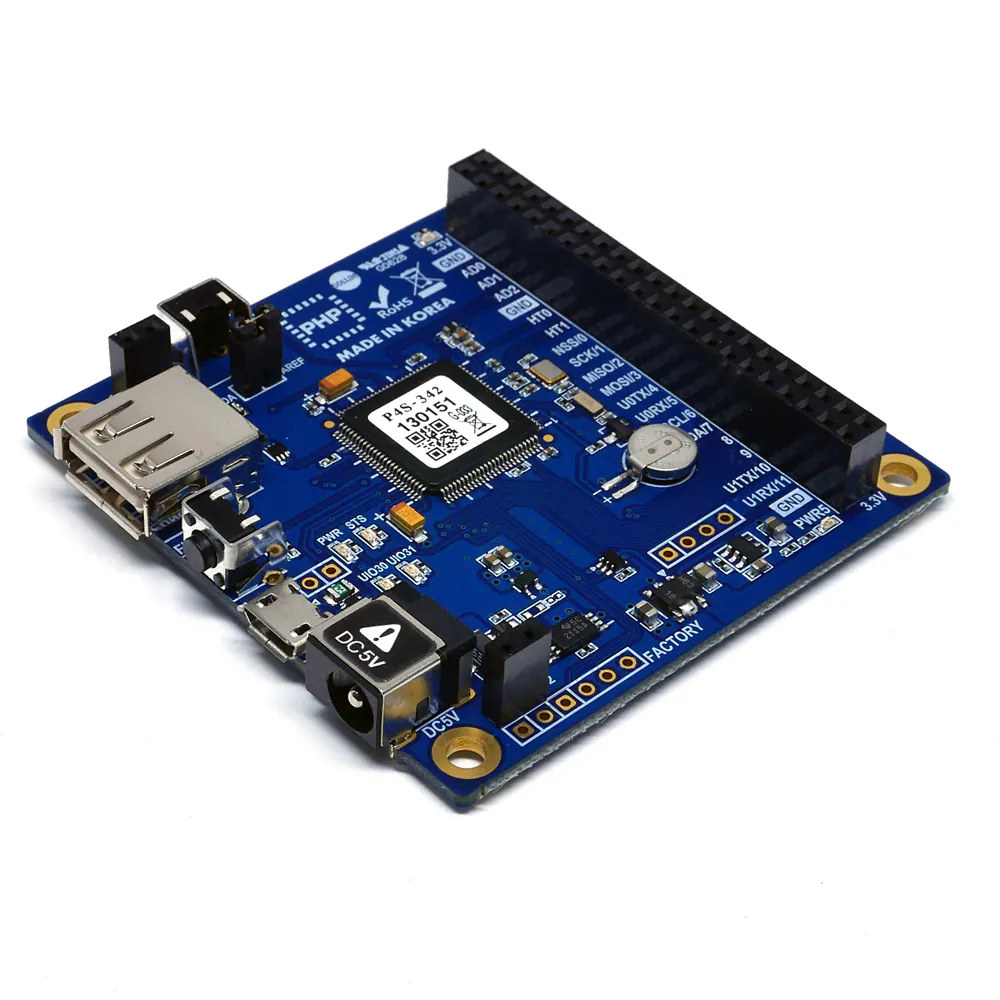PHPoC Blue
×1PHPoC DC Motor Controller (S-type or T-type)
×1
 DC Motor with encoder
×1

## Schematics

### plate_C53AFuzJEn.png

Background Image (plate.png)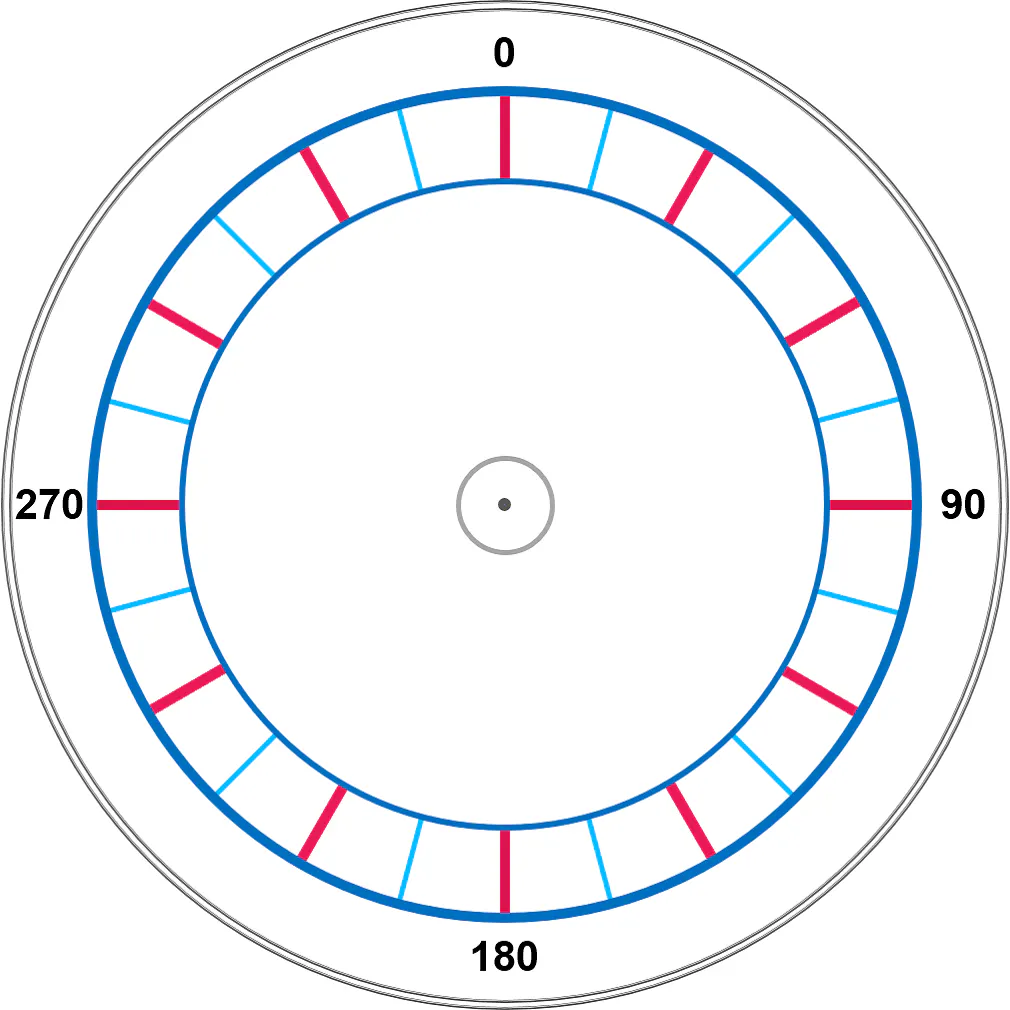### Needle Image (needle.png)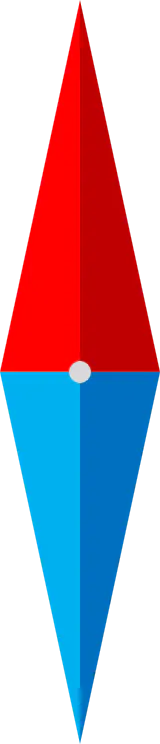### Wiring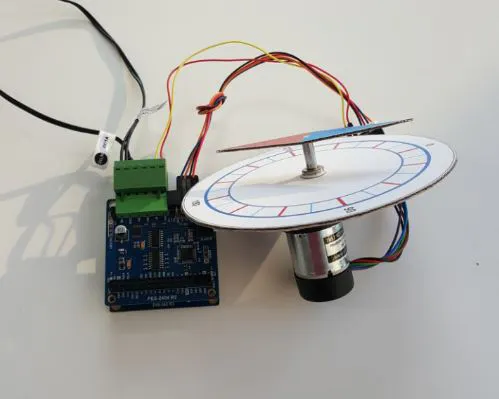## Code

PHP
This is main task, which run in infinite loop. It needs to use PID library from this link https://www.hackster.io/phpoc_man/pid-controller-auto-tuning-library-and-example-for-dc-motor-bc028f
```<?php
include_once "/lib/sd_340.php";
include_once "/lib/sd_spc.php";
include_once "/lib/sn_tcp_ws.php";
include_once "vc_pid.php";

\$sid = 14;
\$dc_id = 1;
\$pwm_period = 10000; //10000 us

st_free_setup(0, "us");
ws_setup(0, "dc_motor_rotate", "csv.phpoc");

spc_reset();
spc_sync_baud(460800);
spc_request_dev(\$sid, "dc\$dc_id pwm set period \$pwm_period");
spc_request_dev(\$sid, "dc\$dc_id enc set psr 64");
spc_request_dev(\$sid, "dc\$dc_id pwm set decay slow");
spc_request_dev(\$sid, "dc\$dc_id lpf set freq 5000");

/*----------- check enc pol-------------- */
\$pwm = 0; \$pos = 0;
while(!\$pos)
{
\$pwm += 10;
spc_request_dev(\$sid, "dc\$dc_id pwm set width \$pwm");
\$pos = (int)spc_request_dev(\$sid, "dc\$dc_id enc get pos");
usleep(1000);
}

if(\$pos < 0)
spc_request_dev(\$sid, "dc\$dc_id enc set pol -");

spc_request_dev(\$sid, "dc\$dc_id pwm set width 0");

/*-----------PID parameters-----------*/
\$pid = array(0, 0, 0, 0, 0, 0, 0, 0, 0, 0, 0, 0);

//\$pid[INDEX_KP_GAIN]	= 0; // manually set by user or automatically set by auto-tuning function
//\$pid[INDEX_KI_GAIN]	= 0; // manually set by user or automatically set by auto-tuning function
//\$pid[INDEX_KD_GAIN]	= 0; // manually set by user or automatically set by auto-tuning function
\$pid[INDEX_INT_TERM]	= 0; // initial value
\$pid[INDEX_PRE_ERROR]	= 0; // initial value
\$pid[INDEX_INT_MAX]  	= 2000; // recommended vaule <= 100% of pwm width for position control
\$pid[INDEX_INT_MIN]	    = -2000; // opposite of upper limit
\$pid[INDEX_PRE_TIME]    = st_free_get_count(0); //current time
\$pid[INDEX_SAMPLE_TIME]	= 10000; // in micro-second
\$pid[INDEX_PID_TYPE]	= PID_TYPE_POS; // position control
\$pid[INDEX_PID_SCALE]	= 5; // depending on motor, should test manually to have the best value
\$pid[INDEX_PWM_PERIOD]	= \$pwm_period;

\$setpoint = 0; //desired position

/*----------- PID auto-tuning-------------- */
dc_pid_tune_start();
while(!dc_pid_tune_loop(\$sid, \$dc_id, \$pid))
usleep(10000);

spc_request_dev(\$sid, "dc\$dc_id enc set pos 0");

/*----------- loop -----------*/
while(1)
{
dc_pid_loop(\$sid, \$dc_id, \$setpoint, \$pid);

\$rwbuf = "";

if(ws_state(0) == TCP_CONNECTED)
{

if(\$rlen)
{
\$target_angle = (float)\$rwbuf;
\$setpoint = (int)(\$target_angle / 360.0 * (13 * 100 * 4));
}

//\$pos = (int)spc_request_dev(\$sid, "dc\$dc_id enc get pos");
//echo "\$pos \$setpoint\r\n";
}
}

?>
```

### index.php

PHP
Web User Interface
```<!DOCTYPE html>
<html>
<title>PHPoC / <?echo system("uname -i")?></title>
<meta name="viewport" content="width=device-width, initial-scale=0.7">
<style>
body { text-align: center; background-color: whiite;}
canvas {
background-color: white;
background: url(plate.png) no-repeat;
background-size: contain;}
}
</style>
<script>
var CANVAS_WIDTH, CANVAS_HEIGHT;
var PIVOT_X, PIVOT_Y;
var PLATE_WIDTH = 1010.0, PLATE_HEIGHT = 1010.0; // size of background image
var NEEDLE_WIDTH = 160, NEEDLE_HEIGHT = 744; // size of needle image
var RATIO;
var plate_angle = 0;
var needle_img = new Image();
var click_state = 0;
var last_angle_pos = 0;
var mouse_xyra = {x:0, y:0, r:0.0, a:0.0};
var ws;

needle_img.src = "needle.png";

function init()
{
CANVAS_WIDTH = window.innerWidth - 50;
CANVAS_HEIGHT = window.innerHeight - 50;

if(CANVAS_WIDTH < CANVAS_HEIGHT)
CANVAS_HEIGHT = CANVAS_WIDTH;
else
CANVAS_WIDTH = CANVAS_HEIGHT;

PIVOT_X = CANVAS_WIDTH/2;
PIVOT_Y = CANVAS_HEIGHT/2;

RATIO = CANVAS_WIDTH / PLATE_WIDTH;

NEEDLE_WIDTH = Math.round(NEEDLE_WIDTH * RATIO);
NEEDLE_HEIGHT = Math.round(NEEDLE_HEIGHT * RATIO);
//needle_img.width = NEEDLE_WIDTH;
//needle_img.height = NEEDLE_HEIGHT;

var dc_motor = document.getElementById("dc_motor");

dc_motor.width = CANVAS_WIDTH;
dc_motor.height = CANVAS_HEIGHT;

var ctx = dc_motor.getContext("2d");

ctx.translate(PIVOT_X, PIVOT_Y);

rotate_plate(0);

ws = new WebSocket("ws://<?echo _SERVER("HTTP_HOST")?>/dc_motor_rotate", "csv.phpoc");
document.getElementById("ws_state").innerHTML = "CONNECTING";

ws.onopen  = function(){ document.getElementById("ws_state").innerHTML = "OPEN" };
ws.onclose = function(){ document.getElementById("ws_state").innerHTML = "CLOSED"};
ws.onerror = function(){ alert("websocket error " + this.url) };

ws.onmessage = ws_onmessage;
}
function ws_onmessage(e_msg)
{
e_msg = e_msg || window.event; // MessageEvent

plate_angle = Number(e_msg.data);

rotate_plate(plate_angle);
}
function rotate_plate(angle)
{
var dc_motor = document.getElementById("dc_motor");
var ctx = dc_motor.getContext("2d");

ctx.clearRect(-PIVOT_X, -PIVOT_Y, CANVAS_WIDTH, CANVAS_HEIGHT);
ctx.rotate(-angle / 180 * Math.PI);

ctx.drawImage(needle_img, 0, 0, needle_img.width, needle_img.height, -NEEDLE_WIDTH/2, -NEEDLE_HEIGHT/2, NEEDLE_WIDTH, NEEDLE_HEIGHT);

//ctx.drawImage(needle_img, -NEEDLE_WIDTH/2, -NEEDLE_HEIGHT/2);

ctx.rotate(angle / 180 * Math.PI);

debug = document.getElementById("debug");
debug.innerHTML = plate_angle.toFixed(1);
}
function check_update_xyra(event, mouse_xyra)
{
var x, y, r, a;
var min_r, max_r, width;

if(event.touches)
{
var touches = event.touches;

x = (touches.pageX - touches.target.offsetLeft) - PIVOT_X;
y = PIVOT_Y - (touches.pageY - touches.target.offsetTop);
}
else
{
x = event.offsetX - PIVOT_X;
y = PIVOT_Y - event.offsetY;
}

/* cartesian to polar coordinate conversion */
r = Math.sqrt(x * x + y * y);
a = Math.atan2(y, x);

mouse_xyra.x = x;
mouse_xyra.y = y;
mouse_xyra.r = r;
mouse_xyra.a = a;

return true;
else
return false;
}
function mouse_down()
{
if(event.touches && (event.touches.length > 1))
click_state = event.touches.length;

if(click_state > 1)
return;

if(check_update_xyra(event, mouse_xyra))
{
click_state = 1;
last_angle_pos = mouse_xyra.a / Math.PI * 180.0;
}
}
function mouse_up()
{
click_state = 0;
}
function mouse_move()
{
var angle_pos, angle_offset;

if(event.touches && (event.touches.length > 1))
click_state = event.touches.length;

if(click_state > 1)
return;

if(!click_state)
return;

if(!check_update_xyra(event, mouse_xyra))
{
click_state = 0;
return;
}

angle_pos = mouse_xyra.a / Math.PI * 180.0;

if(angle_pos < 0.0)
angle_pos = angle_pos + 360.0;

angle_offset = angle_pos - last_angle_pos;
last_angle_pos = angle_pos;

if(angle_offset > 180.0)
angle_offset = -360.0 + angle_offset;
else
if(angle_offset < -180.0)
angle_offset = 360 + angle_offset;

plate_angle += angle_offset;

rotate_plate(plate_angle);

ws.send(plate_angle.toFixed(4) + "\r\n");

event.preventDefault();
}
</script>

<body>

<h2>
Smart Expansion / DC Motor Rotate<br>
<br>

<canvas id="dc_motor"></canvas>

<p>
WebSocket : <span id="ws_state">null</span><br>
Angle : <span id="debug">0</span>
</p>
</h2>

</body>
</html>
```

## Credits

### phpoc_man

62 projects • 335 followers